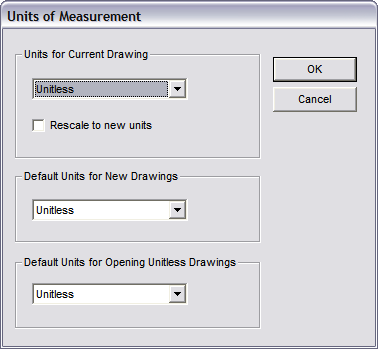# Units of MeasurementLets you specify the base units of measurement for three sets of circumstances:

Rescale to New Units: This causes objects to be correctly sized when the units are changed. If this option is off an object 1cm is changed to 1mm or 1in when the unit type is changed. If the option is on the 1cm object would be converted to a 10mm or 2.54in object.

Units for Current Drawing: Sets (or changes) the default base units for the current drawing. Changing the current drawing’s base units does not affect the numeric value of the distance between two points - in other words, if you switch from inches to miles, the length of what was originally a 10-inch line is now 10 miles; no attempt is made to scale the drawing from the original base unit to the new one.

Default Units for New Drawings: The default base unit that will be used for any new drawings you create.

Default Units for Opening Unitless Drawings: Affects the way unitless symbols and blocks are imported into the drawing. When loading a unitless symbol or block, the imported object’s base units are assumed to be the same as those specified by this setting.

If a unit-based symbol or block is loaded or merged into a unit-based drawing, the symbol’s or block’s base units are converted to those of the host drawing, so that the symbol is imported at the correct “real-world” size. When loading a unit-based symbol or block into a unitless drawing, the symbol or block is also assumed to be unitless (for example, a distance of 10 base units in the symbol becomes a distance of 10 in the unitless drawing).

When entering a distance in an edit box, such as the DX, DY, or DZ fields in Point Relative, a plain decimal number is assumed to be in the current units of measurement. If a value is entered in feet-and-inches formats, such as 3’ 2”, then that distance is converted to the current base unit of measurement. If the current base unit is inches, for example, an entry of 3’ 2” becomes 38 inches; if the current base unit is metered, the entry is converted to 0.9652 meters. If the drawing is unitless, the base unit is implicitly treated as feet anytime a feet-and-inches distance entry is specified (in other words, the example distance entry of 3’ 2” is converted to 3.166666667 units.)

Dimension commands will normally work in the current base unit, so long as a decimal or fractional text format is chosen for the dimension command. However, if a feet-and-inches text format is chosen, then the displayed distance will be converted from the current base unit to feet-and-inches. If feet- and-inches dimension text format is chosen in a unitless drawing, the distance is calculated as though the base unit were feet.